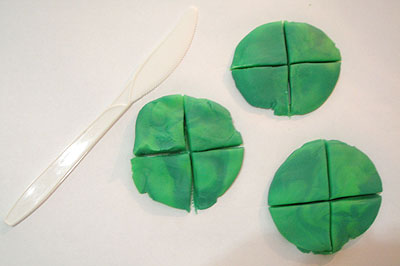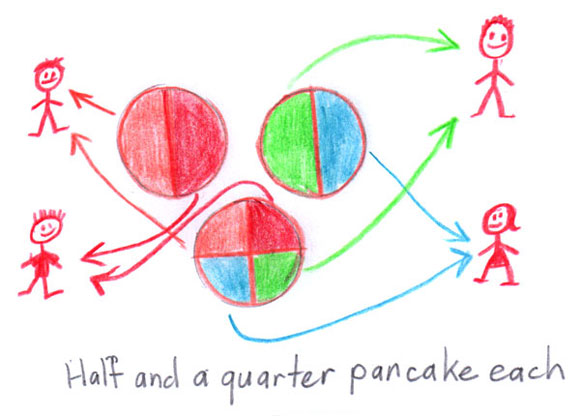Home > Fractions > Activities > Dividing pancakes

# Dividing pancakes

Students solve problems about sharing pancakes equally among people. The numbers are selected to match the appropriate fractions for the year level.

'Share three pancakes among four people' is a year 3 example that requires working with quarters.

'Share nine pancakes among 12 people' is a year 6 example that could involve a variety of fractions, including twelfths.

Students use modelling dough to represent pancakes which they cut with plastic knives into (estimated) equal parts.'Pancakes' divided into quarters.

They record the process through sharing diagrams.A student’s sharing diagram.

The solution strategies should be displayed and discussed, focussing attention on the equivalence of various forms on the answer. Year 3 students should recognise that $$\frac{1}{2}$$ and $$\frac{1}{4}$$ together are the same as $$\frac{3}{4}$$.

Year 5 and 6 students should be able to find several different solution strategies and gradually recognise the relationship between the division task and the resulting fraction.
For example, 9 ÷ 12 = $$\frac{9}{12}$$ which is $$\frac{3}{4}$$.

At this point, the physical modelling of the problem should become unnecessary.